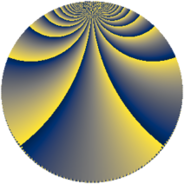# Properties

 Label 1344.4.sLevel $1344$ Weight $4$ Character orbit 1344.s Rep. character $\chi_{1344}(239,\cdot)$ Character field $\Q(\zeta_{4})$ Dimension $288$ Sturm bound $1024$

# Related objects

## Defining parameters

 Level: $$N$$ $$=$$ $$1344 = 2^{6} \cdot 3 \cdot 7$$ Weight: $$k$$ $$=$$ $$4$$ Character orbit: $$[\chi]$$ $$=$$ 1344.s (of order $$4$$ and degree $$2$$) Character conductor: $$\operatorname{cond}(\chi)$$ $$=$$ $$48$$ Character field: $$\Q(i)$$ Sturm bound: $$1024$$

## Dimensions

The following table gives the dimensions of various subspaces of $$M_{4}(1344, [\chi])$$.

Total New Old
Modular forms 1568 288 1280
Cusp forms 1504 288 1216
Eisenstein series 64 0 64

## Trace form

 $$288q + O(q^{10})$$ $$288q + 48q^{19} - 264q^{27} + 1200q^{39} - 864q^{43} + 14112q^{49} + 576q^{55} + 1824q^{61} - 1632q^{67} + 3304q^{75} - 480q^{85} + 2576q^{87} + 4272q^{93} + 5312q^{99} + O(q^{100})$$

## Decomposition of $$S_{4}^{\mathrm{new}}(1344, [\chi])$$ into newform subspaces

The newforms in this space have not yet been added to the LMFDB.

## Decomposition of $$S_{4}^{\mathrm{old}}(1344, [\chi])$$ into lower level spaces

$$S_{4}^{\mathrm{old}}(1344, [\chi]) \cong$$ $$S_{4}^{\mathrm{new}}(48, [\chi])$$$$^{\oplus 6}$$$$\oplus$$$$S_{4}^{\mathrm{new}}(192, [\chi])$$$$^{\oplus 2}$$$$\oplus$$$$S_{4}^{\mathrm{new}}(336, [\chi])$$$$^{\oplus 3}$$